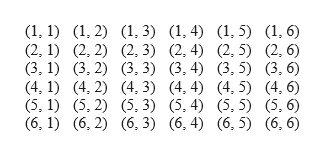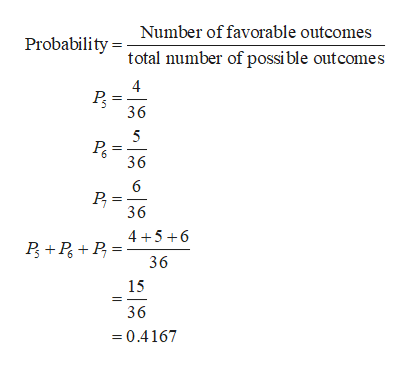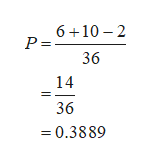Two dice are rolled.  Find the probability of gettingA. A sum of 5,6,7B. Doubles or sum of 6 or 8C. A sum greater than 8 or less than 3D Based on the answers to parts a, b, c which is least likely to occur? explain why?

Question

Two dice are rolled.  Find the probability of getting

A. A sum of 5,6,7

B. Doubles or sum of 6 or 8

C. A sum greater than 8 or less than 3

D Based on the answers to parts a, b, c which is least likely to occur? explain why?

Step 1

Sample space for rolling two dice:

It is given that two dice are rolled. The number of possible outcomes for rolling two dice is 36. The sample space is as follows.help_outlineImage Transcriptionclose(1, 1) (, 2) (, 3) (1,4) (1,5) (1, 6) (2, 1) (2, 2) (2, 3) (2,4) (2.5) (2.6) (3, 1) (3, 2) 3. 3) (3,4) (3, 5) (3. 6) (4, 1 (4, 2) (4, 3) (4, 4) (4, 5) (4,6) (5, 1) (5, 2) (5, 3) (5, 4) (5, 5) (5,6) (6, 1) (6, 2) (6, 3) (6,4) (6, 5) (6, 6) fullscreen
Step 2

A. Probability of getting a sum of 5,6,7:

The probability of getting a sum of 5,6,7 is P5 + PP7

The possible outcomes for which the pair sum is 5 in the total of 36 outcomes are (1, 4), (2, 3), (3, 2), (4, 1). Thus, there are 4 favorable outcomes for which the pair sum is 5.

The possible outcomes for which the pair sum is 6 in the total of 36 outcomes are (1, 5), (2, 4), (3, 3), (4, 2), (5, 1). Thus, there are 5 favorable outcomes for which the pair sum is 6.

The possible outcomes for which the pair sum is 7 in the total of 36 outcomes are (1, 6), (2, 5), (3, 4), (4, 3), (5, 2), (6, 1). Thus, there are 6 favorable outcomes for which the pair sum is 7.

The probability of getting a sum of 5,6,7 is 0.4167 from the calculations given below.help_outlineImage TranscriptioncloseNumber of favorable outcomes Probability tal number of possible outcomes 4 36 36 6 36 4 56 36 15 36 =0.4167 fullscreen
Step 3

B. Probability of getting doubles or sum of 6 or 8 Probability of getting doubles or sum of 6 or 8:

The possible outcomes of getting doubles are (1, 1), (2, 2), (3, 3), (4, 4), (5, 5), and (6, 6). Thus, there are 6 favorable outcomes.

The possible outcomes of getting a sum of 6 or 8 are (1, 5), (2, 4), (2, 6), (3, 3), (3, 5), (4, 2...help_outlineImage Transcriptionclose6 10 2 P = 36 14 36 =0.3889 fullscreen

Want to see the full answer?

See Solution

Want to see this answer and more?

Our solutions are written by experts, many with advanced degrees, and available 24/7

See Solution
Tagged in

Other